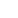Share

# <3

11 September 2021 year at 20:09

time left:

<3<3<3<3<3<3<3<3<3<3<3<3<3<3<3<3<3<3<3<3<3<3<3<3<3<3<3<3<3<3<3

## THE MOST ACTIVE USERS

••••••